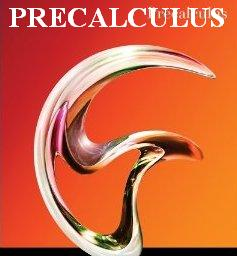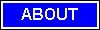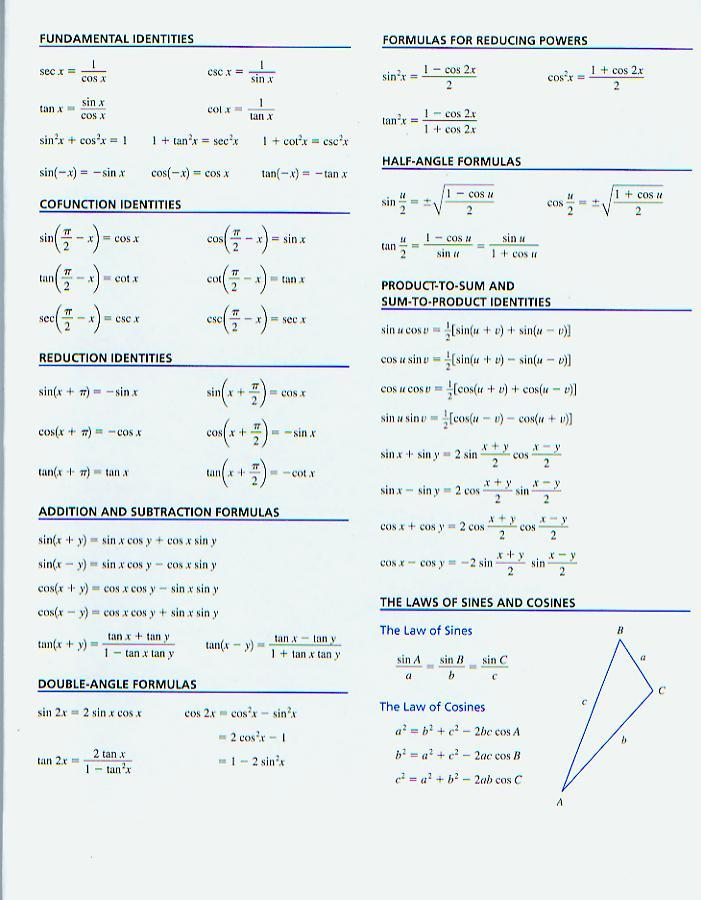M A T H   T U T O R I N G
ONE-ON-ONE
TEST PREPARATION917.392.7004    email: mathtutor255@yahoo.com

FUNCTIONS CAN BE CATEGORIZED, and the simplest type is a polynomial function. We will define it below. We begin with vocabulary. 1. When numbers are added or subtracted, they are called terms. This -- 4x˛ + 7x ? 8 -- is a sum of three terms. (In algebra, we speak of a "sum," even though a term may be subtracted.) When numbers are multiplied, they are called factors. This -- 1. (x + 1)(x + 2)(x + 3) -- is a product of three factors. 2. A variable is a symbol that takes on different values. A value is a number. Thus if x is the variable and has the value 4, then 5x + 1 has the value 21. 3. A constant is a symbol that has a single value. In other words, a constant is a symbol whose value we know. Domain and range The natural domain of any polynomial function is ? < x < . x may take on any real value. Consider the graphs of y = x˛ , and y = xł. Problem 7. Let f(x) be the function with the given, restricted domain. Describe its range. (If you are not viewing this page with Internet Explorer 6, then your browser may not be able to display the symbol ?, "is less than or equal to;" or ?, "is greater than or equal to.") a) f(x) = x˛, ?3 ? x ? 3 0 ? y ? 9. y goes from a low of 0 (at x = 0) to a high of 9 (at both ?3 and 3). b) f(x) = x3, ?3 ? x ? 3 ?27 ? y ? 27. y goes from a low of ?27 (at x = ?3) to a high of 27 (at x = 3). c) f(x) = x4, ?2 ? x ? 1 0 ? y ? 16. y goes from a low of 0, at x = 0, to a high of 16, at xsitemap